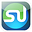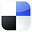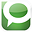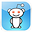# Using the Hypothesis Test in Excel To Find Out If Your Advertising Really Worked

This article will explain how to use Excel to perform a Hypothesis Test to analyze an advertising campaign. Specifically, we will show how to use Excel to perform One-Tailed, Two-Sample, Paired Hypothesis Test of Mean to determine whether an advertising campaign improved really sales.

This Hypothesis Test tests the Null Hypothesis that the advertising campaign did not improve sales.

The advantages of statistical analysis in Excel to solve business statistics problems is that most problems can be solved in just one or two steps and there is no more need to look anything up on Normal Distribution tables.

Here is the problem:

Problem: Determine with 95% certainty whether an advertising campaign increased average daily sales to our large dealer network. Before and After samples of average daily sales were taken with at least 30 dealers.

Here is the Before and After data for all of the dealers:

Before we begin solving this problem, we need to know whether we are dealing with normally distributed data. If the data is not normally distributed, we have to use nonparametric statistical tests to solve this problem.

Always Test for Normality First

Normality tests should be performed on the before and after sales data. Both data sets must be normally distributed to perform the well-known hypothesis test that is based upon the underlying data being normally distributed. This blog has numerous articles about how to perform normality testing and nonparametric testing if the data is not normally distributed.

The MOST Important Step

Determine What Type of Hypothesis

Test You Will Perform﻿

1) Hypothesis Test of Mean or Proportion?

We know that this is a test of mean and not proportion because each individual sample taken can have a wide range of values: Any sales sample measurement from 90 to 250 is probably reasonable.

2) One or Two-Tailed Hypothesis Test?

We know that this is a one-tailed test because we are trying to determine if the "After Data" mean sales is larger than the "Before Data" mean sales, not whether the mean sales are merely different.

3) One or Two-Sample Hypothesis Test?

We know that two samples need to be taken because no data is initially available.

4) Paired or Unpaired Hypothesis Test?

This is paired data because each set of "Before" and "After" data came from the same object.

In this case, we are performing a One-Tailed, Two-Sample, Paired Hypothesis Test of Mean to determine whether an advertising campaign improved really sales. We will do this test in Excel. It is extremely important to establish the type of Hypothesis test. Each type of Hypothesis test uses a slightly (or very) different methodology and set of formulas.
In this case, the yellow-highlighted column represents the difference between the Before and After sample of each data pair. The Hypothesis test will be performed on that column of data.

Above is the data sample:

Paired Hypothesis Tests involve taking Before and After samples from the same large number (n is greater than 30) of objects and performing a Hypothesis test on the differences between the Before and After samples.

Initial Parameters Needed

Before we can begin the Hypothesis test, we need to calculate the following parameters of variable x:

Sample size - Use Excel function COUNT

Sample mean
- Use Excel function AVERAGE

Sample standard deviation - Use Excel function STDEV

Sample standard error = (Sample standard deviation) / SQRT (Sample size)

Difference Data

We need to make the following calculations on the data that represents the difference between the Before and After data:

xavg = "Difference Data" sample average = 9.60
Sxavg = Sample standard error = 1.11

n = "Difference Data" Sample size = 30

α = Level of Significance = 0.05  because there is a 5% max chance of error allowed.

Therefore a 95% Level of Certainty Required

The Four-Step Method That Solves ALL Hypothesis Tests

This problem can be solved using the standard four-step method for Hypothesis testing.

Step 1 - Create the Null and Alternate Hypotheses

The Null Hypothesis normally states that both populations sampled are the same. If the mean sales from both the Before and After Data are the same, then their average difference = 0

The Null Hypothesis states that both Before and After mean sales are the same, which is equivalent to:

Null Hypothesis, H0, is:    xavg = 0

*********************************

The Alternate Hypothesis states that the After Data mean sales is larger, which is equivalent to:

The Alternate Hypothesis, which states that their average difference, xavg, is larger than 0, is as follows:

Alternate Hypothesis, H1 is:  xavg is greater than 0

*********************************

For this one-tailed test, the Alternative Hypothesis states that the value of the distributed variable xavg is larger than the value of 0 stated in the Null Hypothesis,

The Region of Uncertainty will be entirely in the right outer tail.

Note - the Alternative Hypothesis determines whether the Hypothesis test is a one-tailed test or a two-tailed test as follows:

One-tailed test -  (Value of variable) is greater than OR is less than (Constant)

Two-tailed test -  (Value of variable) does not equal (Constant)

Step 2 - Map the Normal Curve

We now create a Normal curve showing a distribution of the same variable that is used by the Null Hypothesis, which is xavg.

The mean of this Normal curve will occur at the same value of the distributed variable as stated in the Null Hypothesis.

Since the Null Hypothesis states that xavg = 0, the Normal curve will map the distribution of the variable xavg with a mean of xavg = 0.

************************************

This Normal curve will have a standard error as just calculated as follows:

Step 3 - Map the Region of Certainty

The problem requires a 95% Level of Certainty so the Region of Certainty will contain 95% of the area under the Normal curve.

We know that this problem uses a one-tailed test with the Region of Uncertainty entirely contained in the outer right tail.

The Region of Uncertainty contains 5% of the total area under the Normal curve. The entire 95% Region of Certainty lies to the left of the 5% Region of Uncertainty, which is entirely contained in the outer right tail.

****************************************

We need to find out how far the boundary of the Region of Certainty is from the Normal curve mean. Calculating the number of standard errors from the Normal curve mean to the outer boundary of the Region of Certainty in the right tail for a one-tailed test is done as follows:

z95%,1-tailed = NORMSINV(1 - α) = NORMSINV(0.95) = 1.65

Excel Note - NORMSINV(x) = The number of standard errors from the Normal curve mean to a point right of the Normal curve mean at which x percent of the area under the Normal curve will be to the left of that point. Additional note - For a one-tailed test, NORMSINV(x) can be used to calculate the number of standard errors from the Normal curve mean to the boundary of the Region of Certainty whether it is in the left or the right tail.

The Region of Certainty extends to the right of the Normal curve mean of xavg = 0 by 1.65 standard errors.

One standard error = sxavg = 1.11, so:

1.65 standard errors = (1.65) * (1.11) = 1.83

The outer boundary of the Region of Certainty has the value = µ + z95%,one-tailed * sxavg

which equals 0 + (1.65) * (1.11) = 0 + 1.83 = 1.83

The point, 1.83, is 1.65 standard errors from the Normal curve mean of xavg = 0

This point, 1.83, is the right boundary of the 95% Region of Certainty on the Normal curve.

a) Critical Value Test

The Critical Value Test is the final test to determine whether to reject or not reject the Null Hypothesis. The p Value Test, described next, is an equivalent alternative to the Critical Value Test.

The Critical Value test tells whether the value of the actual variable, xavg, falls inside or outside of the Critical Value, which is the boundary between the Region of Certainty and the Region of Uncertainty.

If the actual value of the distributed variable, xavg, falls within the Region of Certainty, the Null Hypothesis is not rejected.

If the actual value of the distributed variable, xavg, falls outside of the Region of Certainty and, therefore, into the Region of Uncertainty, the Null Hypothesis is rejected and the Alternate Hypothesis is accepted.

The actual value of the variable xavg = 9.60 and is therefore to the right of (outside of) the outer right Critical Value (1.83), which is the boundary between the Regions of Certainty and Uncertainty in the right tail.

The actual value of the variable xavg is outside the Region of Certainty and therefore outside the Critical Value.

We therefore reject the Null Hypothesis and accept the Alternate Hypothesis which states that average dealer sales have increased after the ad campaign, with a maximum possible error of 5%.

Click On Image To See Larger Version﻿

b) p Value Test

The p Value Test is an equivalent alternative to the Critical Value Test and also tells whether to reject or not reject the Null Hypothesis.

The p Value equals the percentage of area under the Normal curve that is in the tail outside of the actual value of the variable xavg.

For a one-tailed test, if the p Value is larger than α, the Null Hypothesis is not rejected.

For a two-tailed test, if the p Value is larger than α/2, the Null Hypothesis is not rejected.

For a one-tailed test, the Region of Uncertainty is contained entirely in one tail. Therefore the curve area contained by the Region of Uncertainty in that tail equals α.

For a two-tailed test, the Region of Uncertainty is split between both tails. Therefore the curve area contained by the Region of Uncertainty in that tail equals α/2.

The p Value for the actual value of the distributed variable, which in this case is greater than the mean (falls to the right of the mean in the right tail), is:

p Valuexavg = 1 - NORMSDIST( [ xavg - µ ] / sxavg )

Excel note - NORMSDIST(x) calculates the total area under the Normal curve to the LEFT of the point that is x standard errors to the right of the Normal curve mean. Since we are calculating the area to the RIGHT of that point, we use 1 - NORMSDIST.

p Valuexavg = 1 - NORMSDIST((9.60 - 0 ) / 1.11) = 1 - NORMSDIST(9.60/1.11) ≈ 0

The p Value (0) is less than α (0.05), so the Null Hypothesis is rejected and the Alternate Hypothesis is accepted..

For a one-tailed test - When the p Value is less than α, the actual value of the distributed variable falls outside the Region of Certainty and the Null Hypothesis is rejected.

This is the case here.

Click Image To See Larger Version

*****************************************

Using the Hypothesis Test in Excel to Find Out If Your Advertising Worked

If You Like This, Then Share It...1.Strategically pitching and up selling: Sales is an absolute necessity. Any business needs them by snares or law breakers to turn its income cycle. data science course in pune

2.Well, the most on top staying topic is Data Science. Data science is one of the most promising technique in the growing world. I would like to add Data science training to the preference list. Out of all, Data science course in Mumbai
is making a huge difference all across the country. Thank you so much for showing your work and thank you so much for this wonderful article.

3.Just saying thanks will not just be sufficient, for the fantastic lucidity in your writing. I will instantly grab your articles to get deeper into the topic. And as the same way ExcelR also helps organisations by providing data science courses based on practical knowledge and theoretical concepts. It offers the best value in training services combined with the support of our creative staff to provide meaningful solution that suits your learning needs

4.Nice Post...I have learn some new information.thanks for sharing.
ExcelR data analytics course in Pune | business analytics course | data scientist course in Pune

5.Thanks for sharing your valuable information to us, it is very useful.
digital marketing course

6.Such a very useful article. I have learn some new information.thanks for sharing.
data scientist course in mumbai

7.Pretty good post. I just stumbled upon your blog and wanted to say that I have really enjoyed reading your blog posts. Any way I’ll be subscribing to your feed and I hope you post again soon.

Data science course in mumbai

8.Excelr is providing emerging & trending technology training, such as for data science, Machine learning, Artificial Intelligence, AWS, Tableau, Digital Marketing. Excelr is standing as a leader in providing quality training on top demanding technologies in 2019. Excelr`s versatile training is making a huge difference all across the globe. Enable ?business analytics? skills in you, and the trainers who were delivering training on these are industry stalwarts. Get certification on "
best data science course in hyderabad"
and get trained with Excelr.

9.10.This is also a very good post which I really enjoyed reading. It is not every day that I have the possibility to see something like this..
ExcelR data analytics

11.This is a wonderful article, Given so much info in it, These type of articles keeps the users interest in the website, and keep on sharing more ... good luck.

12.Very nice blog here and thanks for post it.. Keep blogging...
ExcelR data science training

13.Attend The PMP Certification in Abu Dhabi From ExcelR. Practical PMP Certification in Abu Dhabi Sessions With Assured Placement Support From Experienced Faculty. ExcelR Offers The PMP Certification in Abu Dhabi.
ExcelR PMP Certification in Abu Dhabi

14.This is a wonderful article, Given so much info in it, These type of articles keeps the users interest in the website, and keep on sharing more ... good luck.
ExcelR data analytics courses

15.Glad to chat your blog, I seem to be forward to more reliable articles and I think we all wish to thank so many good articles, blog to share with us. excelr data science

16.I have to search sites with relevant information on given topic and provide them to teacher our opinion and the article.
ExcelR data analytics courses

17.18.This is a wonderful article, Given so much info in it, These type of articles keeps the users interest in the website, and keep on sharing more ... good luck.
data analytics course mumbai

19.I am a new user of this site so here i saw multiple articles and posts posted by this blog,I curious more interest in some of them hope you will give more information on this topics in your next articles.
Data Scientist course

20.There is obviously a lot to know about this. I think you made some good points in Features also. Keep working, great job!.... data science course Bangalore

21.You actually make it look so easy with your performance but I find this matter to be actually something which I think I would never comprehend. It seems too complicated and extremely broad for me. I'm looking forward for your next post, I’ll try to get the hang of it!
ExcelR Data Analytics Course
Data Science Interview Questions

22.I want to say thanks to you. I have bookmark your site for future updates. ExcelR Data Scientist Course Pune